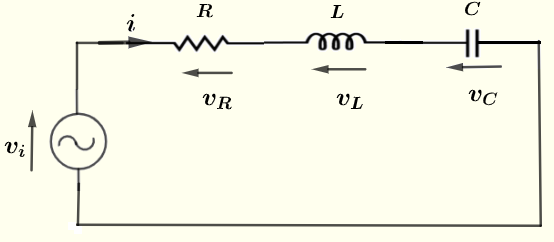# Current and Voltages Calculator for Series RLC circuits

A calculator to calculate the impedance, the current through and voltages across a resistor, a capacitor and an inductor in series. The calculator gives the impedance equivalent to all three components in series, the current and voltages as complex numbers in polar forms. The reference of the phase is such that the phase of the source voltage and assumed to be zero.

  

## Formulae for Impedance, Current and Voltages in series RLC Circuit Used in the Calculator and their Units

We first give the formulas used in the series RLC calculator.The formulas used in the computations of current and voltages in series RLC circuits are presented.
Let $f$ be the frequency, in Hertz, of the source voltage $v_i$ supplying the circuit and define the following parameters used in the calculations
$\omega = 2 \pi f$ , angular frequency in rad/s
$X_C = 1 / (\omega C)$ in $\Omega$ , the reactance of capacitor with capacitance $C$.
$X_L = \omega L$ in $\Omega$ , the reactance of inductor with inductance $L$.
Let $Z$ be the equivalent impedance to the series RLC circuit shown above and write it in complex standard form as follows $Z = R +j (X_L - X_C)$ and in complex polar form as follows $Z = |Z| \; \angle \; \theta$ where the modulus $|Z|$ and argument $\theta$ of $Z$ are given by

Modulus: $|Z| = \sqrt {R^2 + (X_L - X_C)^2 }$ in ohms $(\Omega)$

Argument: $\theta = \arctan \left(\dfrac{X_L - X_C}{R} \right)$ in radians or degrees
Let $v_i = V_0 \cos(\omega t)$
Let $I$, $V_C$, $V_L$ and $V_R$ be the complex forms in polar form of the current $i$, $v_C$, $v_L$ and $v_R$ in the circuit.

$I = \dfrac{V_0}{Z} = \dfrac{V_0}{|Z|} \; \angle \; -\theta$

$V_C = I (- j X_C) = \dfrac{V_0}{|Z|} X_C \; \angle \; -\theta - 90$

$V_L = I (X_L j) = \dfrac{V_0 \cdot X_L}{|Z|} \; \angle \; -\theta + 90$

$V_R = I R = \dfrac{V_0 R}{|Z|} \; \angle \; -\theta$
Note
1) That all the phases are measured taking the phase of $v_i$ as a reference.
2) There is a numerical solution at the bottom of this page for the default values of the source voltage peak value, the resistance, the capacitance, the inductance and the frequency in this calculator.

## Use of the calculator

Enter the source voltage peak value $V_0$, the resistance $R$, the capacitance $C$, the inductance $L$ and the frequency $f$ as positive real numbers with the given units then press "calculate".

 Source Peak Voltage $V_0$ = 10 V Resistance R = 100 mΩ Ω KΩ MΩ Capacitance C = 10 pF nF μF mF F Inductance L = 10 μH mH H Frequency f = 1 GHz MHz kH Hz mHz

## Numerical Example Using the Above Formulas

Let $V_i = 10 ; \angle \; 0$
Frequency $f = 1 \; kHz$ , $C = 10 \; \mu F$ , $L = 10 \; mH$ and $R = 100 \; \Omega$
$X_L = \omega L = 2 \pi f L = 2 \pi 10^3 10^{-2} = 62.83 \; \Omega$
$X_C = \dfrac{1}{\omega C} = \dfrac{1}{2 \pi f C} = \dfrac{1}{2 \pi 10^3 10^{-5} } = 15.92 \; \Omega$
Group imaginary terms
$Z = 100 + j ( 62.83 - 15.92 )$
Simplify
$Z = 100 + j ( 62.83 - 15.92 ) = 100 + 46.91 j$
in phasor form
$Z = 110.45 \; \angle \; 25.13^{\circ}$
$I = \dfrac{V_0}{Z} = \dfrac{V_0}{|Z|} \; \angle \; -\theta$
$\quad \quad = \dfrac{10}{110.45} \; \angle \; - 25.13 ^{\circ} = 0.091 \; \angle \; - 25.13 ^{\circ}$

$V_C = I (- j X_C) = \dfrac{V_0 \cdot X_C}{|Z|} \; \angle \; -\theta - 90$
$\quad \quad = \dfrac{10 \cdot 15.92 }{110.45} \; \angle \; - 25.13 - 90 = 1.441 \; \angle \; -115.13 ^{\circ}$

$V_L = I (X_L j) = \dfrac{V_0 \cdot X_L}{|Z|} \; \angle \; -\theta + 90$
$\quad \quad = \dfrac{10 \cdot 62.83 }{110.45} \; \angle \; - 25.13 + 90 = 5.689 \; \angle \; 64.87 ^{\circ}$

$V_R = I R = \dfrac{V_0 R}{|Z|} \; \angle \; -\theta$
$\quad \quad = \dfrac{10 \cdot 100 }{110.45} \; \angle \; - 25.13 = 9.054 \; \angle \; - 25.13^{\circ}$